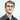# Advent of Code 2019 - Day 5

December 05, 2019

## Day 5: Sunny with a Chance of Asteroids

### Part 1 - Its getting hot in here

1. Add two new instructions to our intcode computer (3) which takes an input value and places it into the given location and (4) which outputs the value at the given position
2. Extend our intcode to support immediate mode in addition to positional, this reads the value directly at a given memory address rather then treating that value as a pointer to the actual value
``````func getAddressOrValue(memory []int, index int, mode int) int {
if mode == 0 {
return memory[memory[index]]
}
return memory[index]
}

func setAddressOrValue(memory []int, index int, value int) {

memory[memory[index]] = value
}

func runInstructions(inputInstructions []int) int {
index := 0
memory := make([]int, len(inputInstructions))
copy(memory, inputInstructions)
input := 5
for index < len(memory)-1 {
opCode := memory[index]
modes := []int{0, 0, 0}
if opCode > 10 {
imOpCode := strconv.Itoa(opCode)
opCode = 0
modeCount := 5 - len(imOpCode)
for i, c := range imOpCode {
intC, err := strconv.Atoi(string(c))
check(err)
if i == len(imOpCode)-1 || i == len(imOpCode)-2 {
opCode += intC
} else {
modes[modeCount] = intC
modeCount++
}

}
fmt.Println(imOpCode, opCode, modes)
}
switch opCode {
case 99:
index = len(memory) - 1
case 1:
index += 4
case 2:
index += 4
case 3:
index += 2
case 4:
index += 2
default:
index = len(memory) - 1
}
}
return memory
}``````

Bit of a hacky solution but it gets the job done, any opcode > 10 is parsed in reverse to determine the mode bits for its parameters.

### Part 2 - Turns out space doesn’t conduct heat very well

For part 2 we need to add 4 instructions to allow us to extend the ships radiative coolers.

1. If true
2. If false
3. Less than
4. Equal
``````case 5:
if leftValue != 0 {
index = rightValue
} else {
index += 3
}
case 6:
if leftValue == 0 {
index = rightValue
} else {
index += 3
}
case 7:
if leftValue < rightValue {
} else {
}
index += 4
case 8:
if leftValue == rightValue {
} else {
}
index += 4``````

### Conclusion

Not a bad couple of problems here, got stuck for a while as I was incorrectly looking at the memory pointer rather then the memory for case 4.

If you have any feedback on my Go code, I’d be happy to hear it!

Github RepoWritten by Daniel Betteridge who lives and works in Perth, WA building useful things.